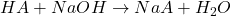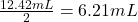## A weak acid is titrated with 0.1236 M NaOH. From the titration curve you determine that the equivalence point occurs after exactly 12.42 mL

Question

A weak acid is titrated with 0.1236 M NaOH. From the titration curve you determine that the equivalence point occurs after exactly 12.42 mL of NaOH have been added. What is the volume of NaOH at the half-equivalence point (a.k.a. the midpoint)

in progress 0
6 months 2021-07-15T22:32:39+00:00 1 Answers 7 views 0

1. Answer: The volume of NaOH required at the half-equivalence point is 6.21 mL

Explanation:

The chemical equation for the reaction of a weak acid with NaOH follows:From the equation, we can say that NaOH and weak acid is present in a 1 : 1 ratio.

We are given:

Volume of NaOH required at equivalence point = 12.42 mL

The volume of NaOH required at half-equivalence point will be =Hence, the volume of NaOH required at the half-equivalence point is 6.21 mL